×#### Thank you for registering.

One of our academic counsellors will contact you within 1 working day.

Click to Chat

1800-1023-196

+91-120-4616500

CART 0

• 0

MY CART (5)

Use Coupon: CART20 and get 20% off on all online Study Material

ITEM
DETAILS
MRP
DISCOUNT
FINAL PRICE
Total Price: Rs.

There are no items in this cart.
Continue Shopping• Complete Physics Course - Class 11
• OFFERED PRICE: Rs. 2,968
• View Details

```Electric Current

Introduction of Electric Current

War of Currents

Relationship between Voltage, Current and Resistance

Kirchhoff’s Law

Types of Current

Direct Current

Alternating Current

Effects of Electric Current

Heating Effect

Magnetic Effect

Summary

Introduction of Electric Current

After Alessandro Volta invented the voltaic pile which was later known as Battery, electric current came much into picture. It used chemical reactions to create an electric potential difference between two different metals which are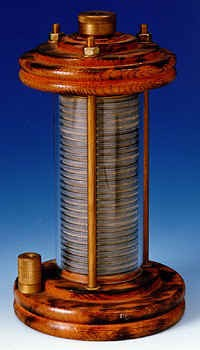known as Electrodes. When two electrodes were connected together, current began to flow. These electrodes are also termed as the terminals. It is possible to connect multiple voltaic cells together by placing wires between their opposite electrodes. We know that the unit of electric potential is named as Volt after his discoveries in the field of electricity.

Michael Faraday has contributed very much in the field of electricity. It includes electromagnetism and electrochemistry. He then invented electric dynamo which is the first model of electric generator, a great contribution in this field. He has developed the faraday cage or the faraday shield which will block the external electric field and is used in various technologies like Ovens, Microwaves, MRI Machines. It is again used to protect electric equipment from lightning.

War of Currents

Thomas Alva Edison’s electric bulb, was one of the finest discovery in the field of electricity. He invented the first commercially practical incandescent light. He bought the patent for the first invented light bulb. He patented a system for electrical distribution. He used DC current to power his system, but it had limitations. These limitation were thrown out by Nikola Tesla who invented the alternating current. He then received patents for electromagnetic motor, AC motor, magnetic motor, electrical transmission of power, electric motor etc.

When the limitations of DC were made public and AC came into picture, Edison launched a propaganda campaign to convince people and merits of Direct current and discredited alternating current. Later the war of currents began. Edison then electrified an elephant by alternating current and tried to prove the danger in using alternating current. However Edison could not win the situation. He could not convince people not to use alternating current. Then Westinghouse who supported Nikola Tesla won the contract to supply electricity to Chicago world’s fair during that time. In the same year Westinghouse was chosen to award the contract to generate power from Niagara Falls by Niagara Falls Power Company.

Electrical current is the flow of charged particles. The electric current flows through a wire the same way as how water moves in a river. The electricity is nothing but the flow of electrons.  When the electric potential varies between two points, it provides energy for the electrons to move from one place to another. In the beginning scientists generated electric current just by rubbing different materials. For the current to flow it requires a circuit. A circuit is a closed loop which consists of wires connected end to end and the electrons flow in same direction. In a circuit, an electric current is defined as the total amount of charge which passes through a wire over a period of time. A circuit consists of conductors (wire), switch, load and a power source.

Relationship between Current, Voltage and Resistance

The unit of electric current is amperes (amps). The ammeter is the instrument which is used to measure the current flowing in a circuit. It can be connected in series with the other elements present in a circuit. The electric current is the rate of flow of the electric charges. Current, I = Q / t, where Q is the charge in coulombs and t is the time taken in seconds. One coulomb of charge passing through the cross section of wire in one second is one ampere of current.

In a circuit electric current is defined as the total amount of charge which passes through a wire over a period of time. The flow of electrons which are negatively charged in one direction is equivalent to the flow of positively charged particles in the opposite direction. In the case of river the strength of the flow of water depends on how far the river flows from high level to a low level. It is similar in the case of electric flow too. As a result of Andre Marie Ampere’s discoveries in this area, the unit of current is named as amp. One amp is equal to one coulomb of charge per second of time.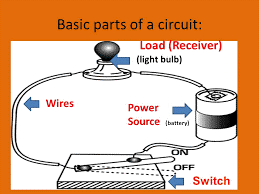Circuit

Voltage can be defined as the difference in electric potential between two points that provides the energy to the electrons to move in a circuit. It is exactly like when a river flows from higher level to a lower level, the electric charge also flows from higher potential or voltage to the lower potential. Volts is the unit of voltage and as discussed earlier was derived from the name Alessandro Volta. Cells or batteries provide the voltage or the potential difference which is required.

Like rocks and branches resist the flow of water, there are elements which obstruct the flow of electrons. This property is called the resistance. The unit of resistance is mentioned in ohms which was named after the scientist Georg Simon ohm. One ohm of resistance lets one volt of potential generating one ampere of current in the circuit. Resistors are used to control the flow of electric current in a circuit. It converts electrical energy into heat and light. Even wires in a circuit possess some resistance. But when comparing them to the resistance of a connected device it can be neglected. By getting rid of natural loss of energy it is possible to reduce the resistance of a material. Thus we can transmit more current.

Power used in a bulb is equal to the current which flows in the circuit times the voltage across the system.

Power P = I V.

Ohm’s Law

Georg Simon ohm described the relationship between voltage, current and resistance and developed the ohm’s law. This law is the basis of electricity. It states that when resistance is constant, the voltage will be directly proportional to the current. The law assumes that the resistance of a material will be constant when the physical conditions like the temperature is constant.

The law states that V = I R, which means voltage is equal to current times resistance. But there are substances for which the resistance will not be a constant and so it alters with current and voltage. For materials known as Ohmic Materials the Law Supports. From the above expression we get I = V / R and R = V / I.

Kirchhoff’s Law

Gustav Kirchhoff developed a set of laws relating to the conservation of current and energy in the electrical circuits. They are KCL (Kirchhoff’s Current Law) which deals with the current flowing in the circuit and KVL (Kirchhoff’s voltage law) which deals with the voltage source present in the circuit.

The first law - Kirchhoff’s Current Law

It states that the algebraic sum of all the currents meeting at a point is zero. The total current that enters a node or junction is equal to the total current or charge leaving the node. This is also known as the Conservation of Charge.Kirchhoff’s current law

The Second Law – Kirchhoff’s Voltage Law

When the current flows in a circuit the magnitude of the current changes in accordance with the product of current and resistance or emf which is connected in the circuit. Thus the law states that the algebraic sum of all voltages within the loop will be equal to zero. This is also known as the Conservation of Energy.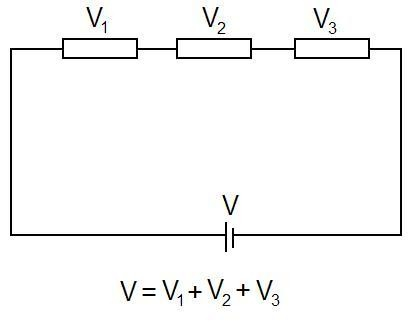Kirchhoff’s voltage law

Types of Current

The electric current is divided into two types. DC – Direct Current and AC - Alternating Current.

Direct Current (DC)

Direct Current is the unidirectional flow of electric charge. The electric charge flows in one direction in DC. This type of current can be produced by the sources such as batteries, power supplies, thermocouples, solar cells etc. It may flow in a conductor like wires. It will also flow through semiconductors, insulators and also through vacuum as in electron. Direct current was known before as Galvanic Current. DC can also be produced from alternating current supply by a rectifier, which contains electromechanical elements or electronic elements and finally allows the current to flow in one direction. Also direct current can also be converted into alternating current with the help of an inverter. DC is used to charge batteries and other power supplies. DC is converted to AC with the help of generators and motors.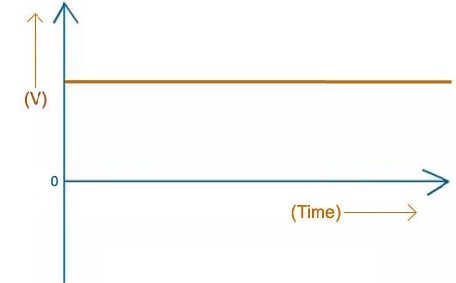Direct current

When a conductor is placed in the presence of magnetic field, there occurs a steady flow of electrons in the same direction. This gives rise to the direct current. It was discovered by conducting a number of experiments by Thomas Edison. The frequency of direct current is zero. In a graph the DC current is a flat line. The load in DC is normally resistive in nature. It is used for electroplating, electrolysis and others.

Alternating Current (AC)

The electric charge in AC changes the direction periodically. Thus the voltage level also gets reversed along with the current. The alternating current can be produced by a device known as alternator. AC current is obtained from AC generator and mains.

The alternating current can be represented in the form of a sine wave. In some applications, it can be also represented in other forms such as square waves or triangular waves. The up curve shows that the current flows in positive direction and the down curve shows that the current flows in negative direction. Examples for alternating current include audio and radio signals. AC typically alternate at higher frequencies than those used in power transmission. It is not much expensive. It was Nikola Tesla who invented the alternating current system and later he developed ac motor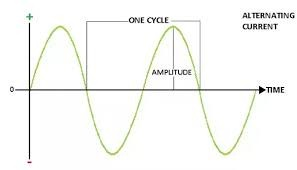Alternating current

The electric current in our house and business world is alternating current. This emerges from the electric power plants that are operated by the electrical companies. It is generated by the generators in the power plants. The cycle of switching the direction is known as the frequency. The frequency of this current is 60 Hertz which means the direction of the current changes sixty times in each second. In ac electricity the voltage is readily changed and thus it is more suitable for long distance transmission when compared to the direct current.

The AC voltages can be transformed to higher or lower voltage levels. This changing of voltages is done with the help of transformers. The current produced is increased to a higher voltage by step up transformers at the power plant and will be decreased to lower voltage by Step down transformer at local areas. So, the Alternating Current (AC) is much safer compared to dc as it can be operated at lower current. The load in ac can be resistive, inductive or even capacitive

Nikolas Tesla placed a conductor in the presence of a rotating magnet and thus it induced a change in the direction of current flow. This in turn produced alternating current. Later Edison’s battery system was replaced by ac generators which was invented by George Westinghouse. The Alternating Current (AC) that reaches at our home is converted to direct current by adaptors.

Effects of Electric Current

The main two effects of electric current is Heating Effect and Magnetic Effect.

Heating Effect

When electric current is passed through an electric bulb, the filament of the bulb gets heated and thus it emits light. This happens due to the heating effect of electric current. When we convert electricity to other energy forms a small amount of energy is converted into heat energy. The heating effect is used in used in many other appliances like electric heater, electric iron, geyser etc. When current passes through a conductor, due to the resistance it offers to the current flow, it generates heat. H = I2 Rt, where H is the heat produced by an element, I is the current passing through the conductor, R is the resistance offered by the conductor and t is the time taken. It is known as Joule’s Law.

Another application for heating effect is the electric fuse. Electric fuse protects the circuit from over flow of current. It consists of a low resistance metallic wire which melts when short circuit or overcurrent occurs. It happens due to the heat generated by large current which flows through it and thus the power supply gets disconnected. After the short circuit the fuse wire should be replaced by a new wire. Fuses are used in AC and DC.Electric fuse

Magnetic Effect

The electric current when passes through a wire produces a magnetic field around the wire. Hans Christian Oersted discovered that the compass needle got deflected when current was passed through a metallic wire. The magnetic effect which is produced by electric current is known as Electromagnetic Effect. The applications are Electric Motor, Loudspeakers, Ammeters, Voltmeters etc.

Summary

Electric current is defined as the flow of charged particle (electrons)

The concept of electromagnetic induction was discovered by Michael faraday. Alessandro Volta invented electric battery and it was first named as voltaic pile. Thomas Alva Edison invented electric bulb.

Current is the total amount of charge which passes through a wire over a period of time. The unit of current in a circuit is amperes (amps). Voltage is the difference in electric potential between two points providing energy to the electrons to move in a circuit. Resistance restricts the flow of electrons in a circuit.

The ohm’s law states that V = I R, where voltage V is in volts, Current I is in amps and resistance R is in ohms.

Kirchhoff’s current law states that the algebraic sum of all the currents meeting at a point is zero. Kirchhoff’s voltage law mentions that the algebraic sum of all voltages within the loop will be equal to zero.

Direct current flows in one direction. It was discovered by Thomas Edison.

The electric charge in AC changes the direction periodically. The alternating current was invented by Nikola Tesla and later he developed ac motor.

When we convert electricity to other energy forms a small amount of energy is converted into heat energy. The magnetic field is produced around a wire when electric current is passed through it.

Watch this Video for more reference

Electric Current
```### Course Features

• 101 Video Lectures
• Revision Notes
• Previous Year Papers
• Mind Map
• Study Planner
• NCERT Solutions
• Discussion Forum
• Test paper with Video Solution